Teardrop Curve
TEARDROP CURVE

Balmoral Software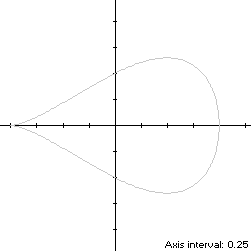A second-order teardrop curve opening to the right is a non-convex x-symmetric closed curve with parametric equations
x(t) = -cos(t)

y(t) = sin(t)sin2(t/2), 0 ≤ t < 2π

The curve follows a clockwise path as t increases, starting from the left cusp at (x(0),y(0)) = (-1,0). Its extreme abscissas occur at the cusp and at (1,0) when t = π. The maximum ordinate of the teardrop curve occurs at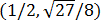when t = 2π/3, so the width x height of its bounding rectangle is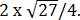### Metrics

We have
x'(t) = sin(t)

y'(t) = [cos(t) - cos(2t)]/2

so by (L1), the perimeter of the teardrop curve is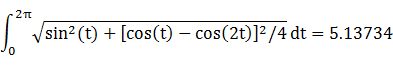and its area is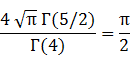By (C1), its centroid abscissa is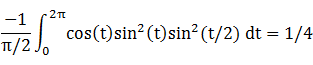(Note: WolframAlpha has reversed the coefficients.)

### Convex Hull

We have
x''(t) = sin(t)

y''(t) = sin(2t) - sin(t)/2,

so by (X1), the teardrop curvature changes sign at (-1,0) since
sin(t)[sin(2t) - sin(t)/2] = cos(t)[cos(t) - cos(2t)]/2
is satisfied for t = 0. Equation (X2) is evaluated with respect to the point (-1,0) as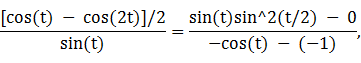which has a solution in the upper half-plane at t = π/2, so the convex hull line segments extend between the points (-1,0) and (x(π/2),±y(π/2)) = (0,±1/2). These line segments are shown in blue in the left diagram below, and each has length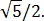By (L1), the perimeter of the convex hull is: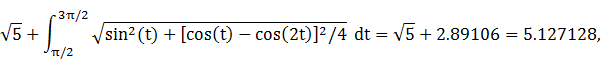which is less than 1% shorter than that of the teardrop curve.

The line segments of the convex hull create an isosceles triangle with its base on the y-axis, having area 1/2. The centroid abscissa of this triangle is the average of its vertex abscissas, or -1/3. By (A1), the remainder of the convex hull has area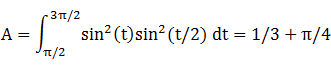By (C1), the associated centroid abscissa is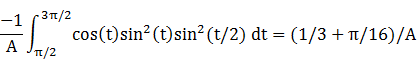The convex hull component metrics can be summarized as follows:
RegionAreaCentroid abscissaProduct
Triangle1/2-1/3-1/6
RemainderA = 1/3 + π/4(1/3 + π/16)/A1/3 + π/16
Total5/6 + π/41/6 + π/16
The area of the convex hull is 1.618732, about 3% larger than that of the teardrop curve. The centroid abscissa of the convex hull is the weighted average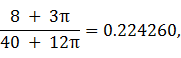which is 1/(20 + 6π) to the left of the centroid of the teardrop curve itself, exactly the same distance as the corresponding value for the piriform curve.

### Incircle

The maximum ordinate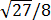of the teardrop curve does not define its inradius since the corresponding abscissa 1/2 is too close to the right edge (1,0), so its incircle is constrained by the right edge. Using z = 1 in Lemma C, we have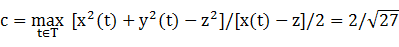and R = |c - z| =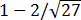. For verification, we have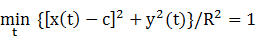### Inellipse

Using z = 1 in Lemma E,
d/dt [x(t) - z]y(t) = d/dt -sin3(t)/2
has a zero at t* = π/2. The corresponding coordinates are
x* = 0

y* = 1/2,

which is the endpoint of a convex hull line segment. We then have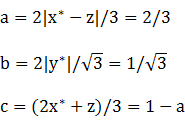For verification,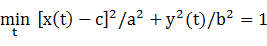### Circumellipse

Using z = -1 in Lemma E,
d/dt [x(t) - z]y(t) = d/dt 2sin4(t/2)sin(t)
has a zero at t* = Arccos(-2/3). The corresponding coordinates are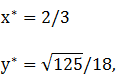from which we haveFor verification,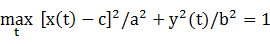### Circumcircle

Since the abscissa extrema are on the x-axis and the bounding rectangle is wider than it is tall, a candidate for the circumcircle has radius R = 1 and center at the origin. For verification, we have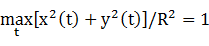### Summary Table

 Perimeter Area Centroid Figure Parameters Incircle R =3.864786 1.188615 (0.384900,0) Inellipse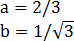3.913233 1.209200 (0.333333,0) Teardrop curve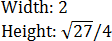5.13734 1.570796 (0.25,0) Convex hull 5.127128 1.618732 (0.224260,0) Circumellipse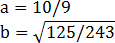5.810712 2.503567 (1.111111,0) Circumcircle R = 1 6.283185 3.141593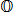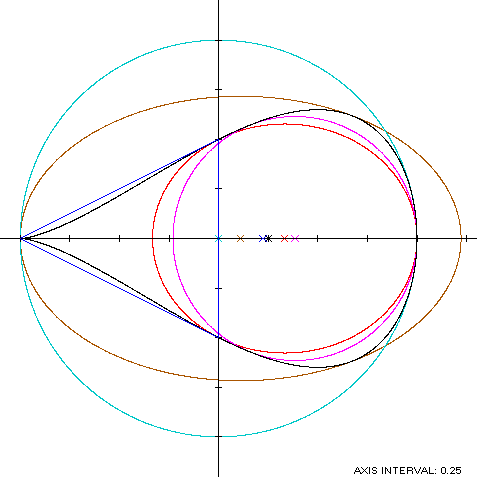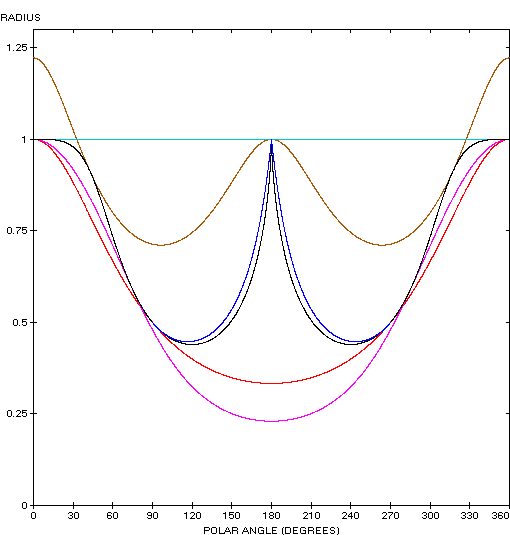The teardrop curve (red) is a member of a group of similarly-shaped figures described on these pages, including (inside to outside) the Tschirnhausen cubic, the right strophoid, the Trisectrix of Maclaurin and the piriform curve: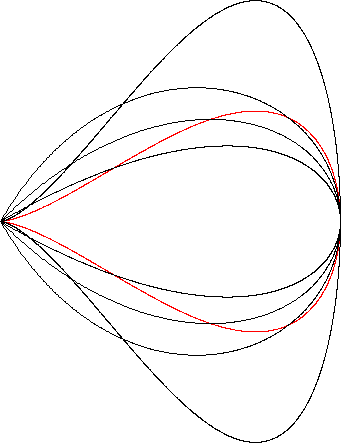### Relationship with the piriform curve

The teardrop curve coincides with the piriform curve after translating its abscissas to the right by 1 and doubling its ordinates. The transformed coordinate functions are
x1(t) = x(t) + 1 = 1 - cos(t)

y1(t) = 2y(t) = 2sin(t)sin2(t/2)

These coordinate functions cannot be directly compared with the corresponding ones for the piriform curve since the rates of travel along the path T (if t is considered a time variable) vary between the two curves. However, the path itself is the same for both curves since x1(t), y1(t) satisfy the cartesian form of the piriform curve:
y2 = x3(2 - x)